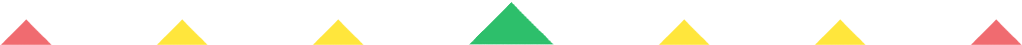Player 1
vs
Player 2
0:00
Opponent history
x
xCorrect
0
Wrong
0
Correct!
Wrong!
Help Sheet for

• 1 = I
• 2 = II
• 3 = III
• 4 = IV
• 5 = V
• 6 = VI
• 7 = VII
• 8 = VIII
• 9 = IX
• 10 = X

• 11 = XI
• 12 = XII
• 13 = XIII
• 14 = XIV
• 15 = XV
• 16 = XVI
• 17 = XVII
• 18 = XVIII
• 19 = XIX
• 20 = XX

• 21 = XXI
• 22 = XXII
• 23 = XXIII
• 24 = XXIV
• 25 = XXV
• 26 = XXVI
• 27 = XXVII
• 28 = XXVIII
• 29 = XXIX
• 30 = XXX

• 31 = XXXI
• 32 = XXXII
• 33 = XXXIII
• 34 = XXXIV
• 35 = XXXV
• 36 = XXXVI
• 37 = XXXVII
• 38 = XXXVIII
• 39 = XXXIX
• 40 = XL

• 41 = XLI
• 42 = XLII
• 43 = XLIII
• 44 = XLIV
• 45 = XLV
• 46 = XLVI
• 47 = XLVII
• 48 = XLVIII
• 49 = XLIX
• 50 = L